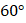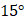# A particle of massmoving with velocity 1 m/s collides perfectly elastically with another stationary particle of mass. If the incident particle is deflected bythe heavy mass will make an anglewith the initial direction ofequal to a)b)c)d)## Question ID - 100218 :- A particle of massmoving with velocity 1 m/s collides perfectly elastically with another stationary particle of mass. If the incident particle is deflected bythe heavy mass will make an anglewith the initial direction ofequal to a)b)c)d)3537

(d)

From conservation of momentum along incident direction(i)

From conservation of momentum along perpendicular direction,(ii)From energy conservation,(iii)

From Eqs. (i), (ii) and (iii), we getNext Question :

In rectangleandAxisandpass through the centre of the rectangle. The moment of inertia is least abouta)b) BC c)d)